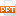# Bài giảng Data Structures & Algorithms - Chương 3: Searching Techniques

To finding out whether a particular element is present in the list.

2 methods: linear search, binary search

The method we use depends on how the elements of the list are organized

unordered list:

linear search: simple, slow

an ordered list:

binary search or linear search: complex, faster15 trang | Chuyên mục: Cấu Trúc Dữ Liệu & Giải Thuật | Chia sẻ: dkS00TYs | Ngày: 08/06/2015 | Lượt xem: 957 | Lượt tải: 1
Tóm tắt nội dung Bài giảng Data Structures & Algorithms - Chương 3: Searching Techniques, để xem tài liệu hoàn chỉnh bạn click vào nút "TẢI VỀ" ở trên
`Click to edit Master title style Click to edit Master text styles Second level Third level Fourth level Fifth level 05/05/2014 ‹#› Data Structures & Algorithms Searching Techniques LINEAR (SEQUENTIAL) SEARCH BINARY SEARCH COMPLEXITY OF ALGORITHMS To finding out whether a particular element is present in the list. 2 methods: linear search, binary search The method we use depends on how the elements of the list are organized unordered list: linear search: simple, slow an ordered list: binary search or linear search: complex, faster LINEAR (SEQUENTIAL) SEARCH How? Proceeds by sequentially comparing the key with elements in the list Continues until either we find a match or the end of the list is encountered. If we find a match, the search terminates successfully by returning the index of the element If the end of the list is encountered without a match, the search terminates unsuccessfully. int lsearch(int arr[], int n, int value) { for(int i=0;i<n;i++) 	if( arr[i] == value) 	 	 return i; 	return -1; } LINEAR (SEQUENTIAL) SEARCH How to run by hand??? average time: O(n) BINARY SEARCH List must be a sorted one We compare the element with the element placed approximately in the middle of the list If a match is found, the search terminates successfully. Otherwise, we continue the search for the key in a similar manner either in the upper half or the lower half. void bsearch(int arr[],int n,int value) { int low,up,mid; low = 0; up = n-1; while(low <= up ) { mid = (low+up)/2; if(arr[mid] == value) 	 return mid; 	 else if(arr[mid] < value) low = mid+1; else up = mid-1; } return -1; } average time: O(log2n) How to run by hand??? int Search (int arr[], int value, int low, int up) { if (low <= up) { int mid = (low + up)/2; if (value == arr[mid]) 	return mid; else if (value < arr[mid]) 	return Search(arr,value,low,mid-1); else return Search(arr,value,mid+1,up); } return -1; } By Recursion COMPLEXITY OF ALGORITHMS In Computer Science, it is important to measure the quality of algorithms, especially the specific amount of a certain resource an algorithm needs Resources: time or memory storage (PDA?) Different algorithms do same task with a different set of instructions in less or more time, space or effort than other. The analysis has a strong mathematical background. The most common way of qualifying an algorithm is the Asymptotic Notation, also called Big O. COMPLEXITY OF ALGORITHMS It is generally written as Polynomial time algorithms, O(1) --- Constant time --- the time does not change in response to the size of the problem. O(n) --- Linear time --- the time grows linearly with the size (n) of the problem. O(n2) --- Quadratic time --- the time grows quadratically with the size (n) of the problem. In big O notation, all polynomials with the same degree are equivalent, so O(3n2 + 3n + 7) = O(n2) Sub-linear time algorithms O(logn) -- Logarithmic time Super-polynomial time algorithms O(n!) ; O(2n) COMPLEXITY OF ALGORITHMS Example1: complexity of an algorithm void func ( int a[], int n ) { cout<< "N = “<< n; for (int i = 0; i < n; i++ ) cout<<a[i]; cout<< n; } 2 * O(1) + O(N) O(N) COMPLEXITY OF ALGORITHMS Example2: complexity of an algorithm void func ( int a[], int n ) { cout<< "N = “<< n; for (int i = 0; i < n; i++ ) 	for (int j=0;j<n;j++) 	 cout<<a[i]<<a[j]; 	for (int i = 0; i < n; i++ ) 	cout<<a[i]; cout<< n; } 2 * O(1) + O(N)+O(N2) O(N2) COMPLEXITY OF ALGORITHMS Linear Search O(n). Binary Search O(log2 N) END `

File đính kèm:

•Chapter 3-Searching Techniques.pptx
Tài liệu liên quan